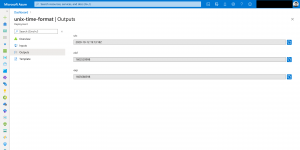Daniel's Tech Blog

Cloud Computing, Cloud Native & Kubernetes

# Unix time format in Azure Resource Manager templates

The Unix time format is represented in seconds elapsed since 01.01.1970 UTC. So, it is different from the standard time format we are used to.

Depending on the Azure service some attributes in the Azure API / ARM template require the Unix time format especially Azure Key Vault keys and secrets.

Those ones have two attributes the not before (nbf) and expiry (exp) date requiring the Unix time format.

Converting the standard time format into the Unix one outside of an ARM template and passing it over as a parameter is the easiest approach.

See the following Bash and PowerShell examples on how to do the conversion.

#### Bash

```#Linux

nbf: date '+%s'

exp: date -d '+2years' '+%s'

#macOS

nbf: date '+%s'

exp: date -v '+2y' '+%s'```

#### PowerShell

```nbf: ([DateTimeOffset](Get-Date)).ToUnixTimeSeconds()

#### Azure Resource Manager templates

If you want doing it natively in ARM templates it gets complicated. As there exists no function for it.

I am saying explicit natively here as you can leverage deployment scripts to do it during the deployment.

The solution you see in a minute is definitely overengineered and based on the utcNow function that is used in the defaultValue of one of the parameters.

```...
"parameters": {
"basetime": {
"type": "string",
"defaultValue": "[utcNow('u')]"
},
"nbfDuration": {
"type": "string",
"defaultValue": "PT0H"
},
"expDuration": {
"type": "string",
"defaultValue": "P2Y"
}
},
...```

Let us start with the first variables section and we just focus on the nbf calculation as the exp one is the same.

```...
"variables": {
//nbf - Unix time converter
"nbfUtc": {
},
...
},
...```

As you see above, we get the date and time in its single components instead as a whole and converting the string into an int value.

```...
"nbfCalculation": {
"yearCalc": "[sub(variables('nbfUtc').year, 1970)]",
"leapYears": "[div(sub(variables('nbfUtc').year, 1970), 4)]",
"monthCalc": "[string(sub(variables('nbfUtc').month, 1))]",
"dayCalc": "[sub(variables('nbfUtc').day, 1)]"
},
...```

Then we calculate how many years have passed and an inaccurate value of how many leap years we had in this time. Same is done for month and days.

```...
"nbfIntermediateCalculation": {
"yearInSeconds": "[add(mul(mul(variables('nbfCalculation').yearCalc, 365), 86400), if(equals(variables('nbfModLeapYear'), 0),mul(variables('nbfCalculation').leapYears, 86400),mul(sub(variables('nbfCalculation').leapYears, 1), 86400)))]",
"monthInSeconds": "[mul (variables(concat('nbf',variables('nbfCalculation').monthCalc)), 86400)]",
"dayInSeconds": "[mul(variables('nbfCalculation').dayCalc, 86400)]",
"hourInSeconds": "[mul(variables('nbfUtc').hour, 3600)]",
"minutesInSeconds": "[mul(variables('nbfUtc').minute, 60)]"
},
...```

Now we convert year, month, day, hour, and minute values into seconds. Considering if we have currently a leap year or not and doing the correct calculation on how many leap years we got since 1970.

For converting the month value correctly, we need the exact amount of days for it. This is done by using the following variables.

```...
"nbfModLeapYear": "[mod(variables('nbfUtc').year, 4)]",
"nbf1": 31,
"nbf2": "[add(31, if(equals(variables('nbfModLeapYear'), 0), 29, 28))]",
"nbf3": "[add(62, if(equals(variables('nbfModLeapYear'), 0), 29, 28))]",
"nbf4": "[add(92, if(equals(variables('nbfModLeapYear'), 0), 29, 28))]",
"nbf5": "[add(123, if(equals(variables('nbfModLeapYear'), 0), 29, 28))]",
"nbf6": "[add(153, if(equals(variables('nbfModLeapYear'), 0), 29, 28))]",
"nbf7": "[add(184, if(equals(variables('nbfModLeapYear'), 0), 29, 28))]",
"nbf8": "[add(215, if(equals(variables('nbfModLeapYear'), 0), 29, 28))]",
"nbf9": "[add(245, if(equals(variables('nbfModLeapYear'), 0), 29, 28))]",
"nbf10": "[add(276, if(equals(variables('nbfModLeapYear'), 0), 29, 28))]",
"nbf11": "[add(306, if(equals(variables('nbfModLeapYear'), 0), 29, 28))]",
"nbf12": "[add(337, if(equals(variables('nbfModLeapYear'), 0), 29, 28))]",
...```

The variable nbfModLeapYear is also used for the calculation for the correct amount of leap years since 1970 in the variable nbfIntermediateCalculation.yearInSeconds.

Finally, we sum up all the values and get the Unix time format.

```...
...```You find the example Azure Resource Manager template on GitHub.

As seen in the screenshot it just does the conversion and provides the value of the utcNow function, the nbf and exp result.

I already provided the feedback to the product group at Microsoft to implement a Unix time format function for ARM templates.

#### Summary

Frankly, you should provide the values directly in Unix time format as parameters or use the deployment script functionality.

The native solution is overengineered and adds additional 70 lines to the variables section of a template. Just having it natively in the ARM template itself.

Posted

in